Next: 4. An Alternative Approach Up: 3. The Box Integration Previous: 3.2 The Diffusion Equation

# 3.3 The Basic Semiconductor Equations

The classic drift-diffusion equations for electrons and holes read3.1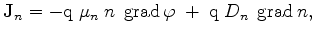(3.62)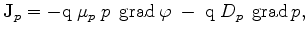(3.63)

with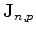the electron or hole current densities,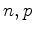the electron or hole concentrations,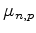and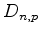the carrier mobilities and diffusivities, and finally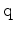the unit charge of the electron (positive). These equations are connected via the recombination rate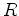(3.64)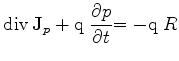(3.65)

and coupled to the already discussed Poisson equation, where the charge is determined via the difference between the ionized impurities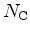and the electron and hole concentrations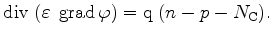(3.66)

The continuity equations (3.64) and (3.65) are converted to integral formulation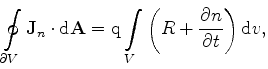(3.67)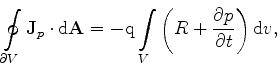(3.68)

which must be satisfied for each Voronoi box of the tessellation. In the discrete form they can be rewritten as in (3.28)-(3.29)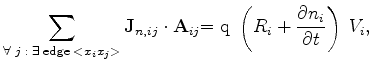(3.69)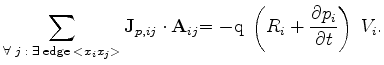(3.70)

The material constants, the electric field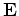and the currents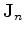and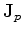are assumed to be constant along each edge and therefore,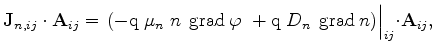(3.71)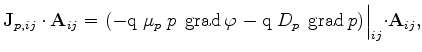(3.72)

where, because of the inner product, only the components of the values along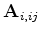or along the edge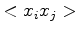remain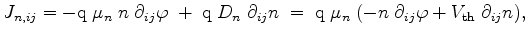(3.73)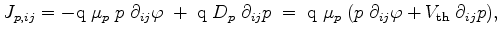(3.74)

with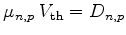and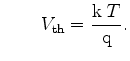(3.75)

The discretization of the transport equations is more crucial, since they are of convection-diffusion type . Because the carrier concentrations change exponentially, a discretization scheme analogously to (3.13) would require an extremely dense mesh. Therefore, a better approximation for the electron and hole concentrations is chosen. As equations (3.73) and (3.74) are defined along the edge, these equations are ordinary differential equations. With the introduction of a local coordinate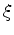along the edge, assumption of constant current density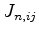, constant mobility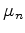, and constant electric field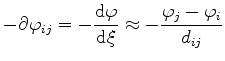(3.76)

along the edge, a linear differential equation of first order is derived, which can be solved for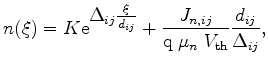(3.77)

with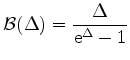and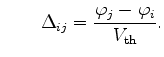(3.78)

The general solution of the differential equation shows two unknowns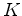andwhich have to be fixed by two boundary conditions (even if it is a differential equation of first order)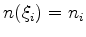and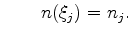(3.79)

The result for the hole current density can be evaluated analogously. Insertion of the boundary conditions and solving forand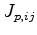delivers(3.80)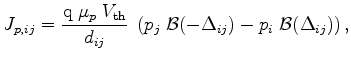(3.81)

which results in the final discrete formulation of the semiconductor equations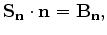(3.82)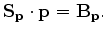(3.83)

Here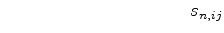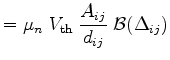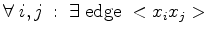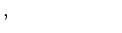(3.84)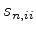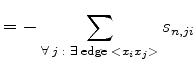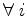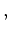(3.85)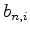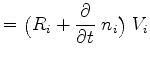(3.86)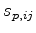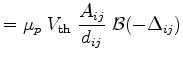(3.87)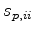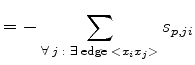, (3.88)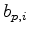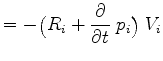. (3.89)

This method is referred as Scharfetter-Gummel discretization . The resulting expressions are linear equation systems in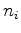or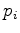where the boundary conditions can be set as in the previous sections. If calculating a non-stationary problem the time discretization can be performed as shown in Section 3.2.

The major difference to the field or diffusion equation is that the system matricesand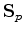are no longer symmetrical, because of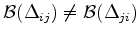. As the semiconductor equations for electrons (3.62) and holes (3.63) depend on the field equation (3.66) and this equation depends on the carrier concentrations itself, the whole differential equation system must be solved. While the dependency is nonlinear in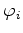, a recursive solution mechanism for solving this system is inevitable. As the coefficients of the matrices are influenced by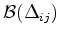, a small potential change will influence the carrier concentrations exponentially, which influences the potential distribution itself. A simple iterative approach, which evaluates each equation back-to-back and reinserts the updated values in the next iteration, does not deliver stable states. Newton or even specially damped Newton algorithms are required .

#### Footnotes

To avoid symbol clashes between grid points and the discrete hole concentration, in this section the grid points are labeled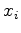.